Hostname: page-component-7d8f8d645b-p72pn Total loading time: 0 Render date: 2023-05-29T23:43:15.979Z Has data issue: false Feature Flags: { "useRatesEcommerce": true } hasContentIssue false

# One-relator groups and algebras related to polyhedral products

Published online by Cambridge University Press:  15 January 2021

## Abstract

We link distinct concepts of geometric group theory and homotopy theory through underlying combinatorics. For a flag simplicial complex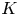$K$, we specify a necessary and sufficient combinatorial condition for the commutator subgroup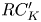$RC_K'$ of a right-angled Coxeter group, viewed as the fundamental group of the real moment-angle complex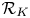$\mathcal {R}_K$, to be a one-relator group; and for the Pontryagin algebra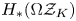$H_{*}(\Omega \mathcal {Z}_K)$ of the moment-angle complex to be a one-relator algebra. We also give a homological characterization of these properties. For$RC_K'$, it is given by a condition on the homology group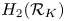$H_2(\mathcal {R}_K)$, whereas for$H_{*}(\Omega \mathcal {Z}_K)$ it is stated in terms of the bigrading of the homology groups of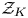$\mathcal {Z}_K$.

Type
Research Article
Information

## Access options

Get access to the full version of this content by using one of the access options below. (Log in options will check for institutional or personal access. Content may require purchase if you do not have access.)

## References

Adams, J. F. and Hilton, P. J.. On the chain algebra of a loop space. Comment. Math. Helv. 30 (1956), 305330.CrossRefGoogle Scholar
Amelotte, S. Connected sums of sphere products and minimally non-Golod complexes. Preprint. (2020) arXiv:2006.16320.Google Scholar
Beben, P. and Wu, J.. The homotopy type of a Poincaré duality complex after looping. Proc. Edinb. Math. Soc. 58 (2015), 581616.10.1017/S0013091515000048CrossRefGoogle Scholar
Buchstaber, V. M. and Panov, T. E.. Toric topology. In Mathematical surveys and monographs, vol. 204 (Providence, RI: American Mathematical Society, 2015), xiv+518 pp.Google Scholar
Dyer, E. and Vasquez, A. T.. Some small aspherical spaces. J. Austral. Math. Soc. 16 (1973), 332352.10.1017/S1446788700015147CrossRefGoogle Scholar
Grbić, J., Panov, T., Theriault, S. and Wu, J.. The homotopy types of moment-angle complexes for flag complexes. Trans. Amer. Math. Soc. 368 (2016), 66636682.CrossRefGoogle Scholar
Grbić, J. and Theriault, S.. The homotopy type of the complement of a coordinate subspace arrangement. Topology 46 (2007), 357396.CrossRefGoogle Scholar
Hatcher, A.. Algebraic topology (Cambridge: Cambridge University Press, 2002).Google Scholar
Iriye, K. and Yano, T.. A Golod complex with non-suspension moment-angle complex. Topol. Appl. 225 (2017), 145163.CrossRefGoogle Scholar
Limonchenko, I. Y.. Families of minimally non-Golod complexes and polyhedral products. Dal'nevost. Mat. Zh. 15 (2015), 222237, (in Russian); arXiv:1509.04302.Google Scholar
Lyndon, R. C.. Cohomology theory of groups with a single defining relation. Ann. of Math. 52 (1950), 650665.CrossRefGoogle Scholar
McGavran, D.. Adjacent connected sums and torus actions. Trans. Amer. Math. Soc. 251 (1979), 235254.CrossRefGoogle Scholar
Panov, T. E. and Ray, N.. Categorical aspects of toric topology. Toric topology, contemporary mathematics, Harada, M. et al. , editors, vol. 460, pp. 293322 (Providence, RI: American Mathematical Society, 2008).CrossRefGoogle Scholar
Panov, T. E. and Veryovkin, Y. A.. Polyhedral products and commutator subgroups of right-angled Artin and Coxeter groups. Sb. Math. 207 (2016), 15821600.CrossRefGoogle Scholar
Veryovkin, Y. A.. Pontryagin algebras of some moment-angle-complexes. Dal'nevost. Mat. Zh. 2016, 923, (in Russian); arXiv:1512.00283.Google Scholar